From 12:00 AM CDT Sunday, October 17 - 11:30 AM CDT Sunday, October 17, ni.com will be undergoing system upgrades that may result in temporary service interruption.

We appreciate your patience as we improve our online experience.

# Concepts

NI Vision 2015 Concepts Help

Edition Date: June 2015

Part Number: 372916T-01

»View Product InfoDownload Help (Windows Only)

## Definition of an Edge

An edge is a significant change in the grayscale values between adjacent pixels in an image. In NI Vision, edge detection works on a 1D profile of pixel values along a search region, as shown in the following figure. The 1D search region can take the form of a line, the perimeter of a circle or ellipse, the boundary of a rectangle or polygon, or a freehand region. The software analyzes the pixel values along the profile to detect significant intensity changes. You can specify characteristics of the intensity changes to determine which changes constitute an edge.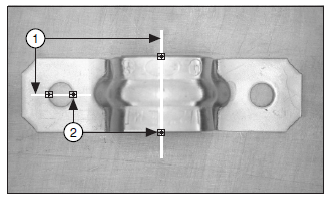1  Search Lines 2  Edges

## Characteristics of an Edge

The following figure illustrates a common model that is used to characterize an edge.

 Gray LevelIntensities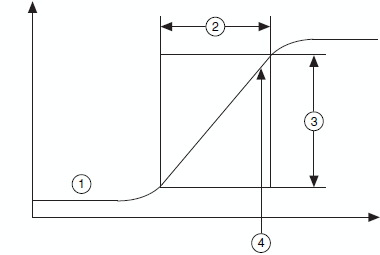SearchDirection
1  Grayscale Profile
2  Edge Length
3  Edge Strength
4  Edge Location

The following list includes the main parameters of this model.

• Edge strength—Defines the minimum difference in the grayscale values between the background and the edge. The edge strength is also called the edge contrast. The following figure shows an image that has different edge strengths. The strength of an edge can vary for the following reasons:
• Lighting conditions—If the overall light in the scene is low, the edges in image will have low strengths. The following figure illustrates a change in the edge strength along the boundary of an object relative to different lighting conditions.
• Objects with different grayscale characteristics—The presence of a very bright object causes other objects in the image with lower overall intensities to have edges with smaller strengths.A B C
• Edge length—Defines the distance in which the desired grayscale difference between the edge and the background must occur. The length characterizes the slope of the edge. Use a longer edge length, defined by the size of the kernel used to detect edges, to detect edges with a gradual transition between the background and the edge.
• Edge location—The x, y location of an edge in the image.
• Edge polarity—Defines whether an edge is rising or falling. A rising edge is characterized by an increase in grayscale values as you cross the edge. A falling edge is characterized by a decrease in grayscale values as you cross the edge. The polarity of an edge is linked to the search direction. The following figure shows examples of edge polarities.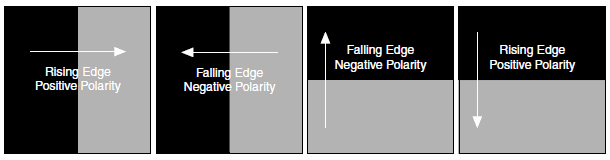## Edge Detection Methods

NI Vision offers two ways to perform edge detection. Both methods compute the edge strength at each pixel along the 1D profile. An edge occurs when the edge strength is greater than a minimum strength. Additional checks find the correct location of the edge. You can specify the minimum strength by using the Minimum Edge Strength or Threshold Level parameter in the software.

### Simple Edge Detection

The software uses the pixel value at any point along the pixel profile to define the edge strength at that point. To locate an edge point, the software scans the pixel profile pixel by pixel from the beginning to the end. A rising edge is detected at the first point at which the pixel value is greater than a threshold value plus a hysteresis value. Set this threshold value to define the minimum edge strength required for qualifying edges. Use the hysteresis value to declare different edge strengths for the rising and falling edges. When a rising edge is detected, the software looks for a falling edge. A falling edge is detected when the pixel value falls below the specified threshold value. This process is repeated until the end of the pixel profile. The first edge along the profile can be either a rising or falling edge. The following figure illustrates the simple edge model.

The simple edge detection method works well when there is little noise in the image and when there is a distinct demarcation between the object and the background.

 Gray LevelIntensities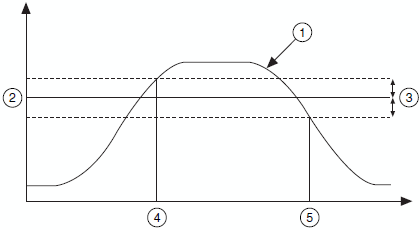Pixels
1  Grayscale Profile
2  Threshold Value
3  Hysteresis
4  Rising Edge Location
5  Falling Edge Location

The edge detection algorithm uses a kernel operator to compute the edge strength. The kernel operator is a local approximation of a Fourier transform of the first derivative. The kernel is applied to each point in the search region where edges are to be located. For example, for a kernel size of 5, the operator is a ramp function that has 5 entries in the kernel. The entries are {–2, –1, 0, 1, 2}. The width of the kernel size is user-specified and should be based on the expected sharpness, or slope, of the edges to be located. The following figure shows the pixel data along a search line and the equivalent edge magnitudes computed using a kernel of size 5. Peaks in the edge magnitude profile above a user-specified threshold are the edge points detected by the algorithm.

 Pixel Intensities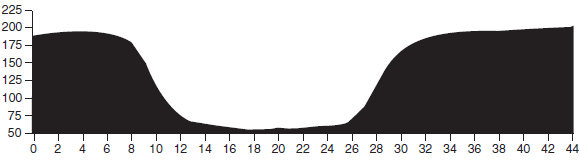Edge Magnitudes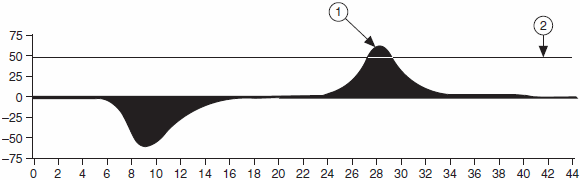1  Edge Location 2  Minimum Edge Threshold

To reduce the effect of noise in image, the edge detection algorithm can be configured to extract image data along a search region that is wider than the pixels in the image. The thickness of the search region is specified by the search width parameter. The data in the extracted region is averaged in a direction perpendicular to the search region before the edge magnitudes and edge locations are detected. A search width greater than 1 also can be used to find a “best” or “average” edge location or a poorly formed object. The following figure shows how the search width is defined.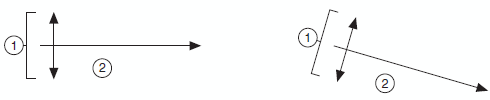1  Search Width 2  Search Line

### Subpixel Accuracy

When the resolution of the image is high enough, most measurement applications make accurate measurements using pixel accuracy only. However, it is sometimes difficult to obtain the minimum image resolution needed by a machine vision application because of limits on the size of the sensors available or the price. In these cases, you need to find edge positions with subpixel accuracy.

Subpixel analysis is a software method that estimates the pixel values that a higher resolution imaging system would have provided. In the edge detection algorithm, the subpixel location of an edge is calculated using a parabolic fit to the edge-detected data points. At each edge position of interest, the peak or maximum value is found along with the value of one pixel on each side of the peak. The peak position represents the location of the edge to the nearest whole pixel.

Using the three data points and the coefficients a, b, and c, a parabola is fitted to the data points using the expression ax2 + bx + c.

The procedure for determining the coefficients a, b, and c in the expression is as follows:

Let the three points which include the whole pixel peak location and one neighbor on each side be represented by (x0, y0), (x1, y1), and (x2, y2). We will let x0 = –1, x1 = 0, and x2 = 1 without loss of generality. We now substitute these points in the equation for a parabola and solve for a, b, and c. The result is

 a = (y0 + y2 – 2y1) 2
 b = (y2 – y0) 2

c = y1, which is not needed and can be ignored.

The maximum of the function is computed by taking the first derivative of the parabolic function and setting the result equal to 0. Solving for x yields

 x = –b 2a

This provides the subpixel offset from the whole pixel location where the estimate of the true edge location lies.

The following illustrates how a parabolic function is fitted to the detected edge pixel location using the magnitude at the peak location and the neighboring pixels. The subpixel location of an edge point is estimated from the parabolic fit.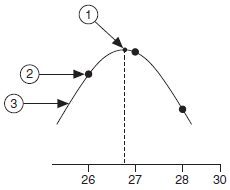1  Interpolated Peak Location     2  Neighboring Pixel 3  Interpolating Function

With the imaging system components and software tools currently available, you can reliably estimate 1/25 subpixel accuracy. However, results from an estimation depend heavily on the imaging setup, such as lighting conditions, and the camera lens. Before resorting to subpixel information, try to improve the image resolution. Refer to system setup and calibration for more information about improving images.

### Signal-to-Noise Ratio

The edge detection algorithm computes the signal-to-noise ratio for each detected edge point. The signal-to-noise ratio can be used to differentiate between a true, reliable, edge and a noisy, unreliable, edge. A high signal-to-noise ratio signifies a reliable edge, while a low signal-to-noise ratio implies the detected edge point is unreliable.

In the edge detection algorithm, the signal-to-noise ratio is computed differently depending on the type of edges you want to search for in the image.

When looking for the first, first and last, or all edges along search lines, the noise level associated with a detected edge point is the strength of the edge that lies immediately before the detected edge and whose strength is less than the user-specified minimum edge threshold, as shown in the following figure.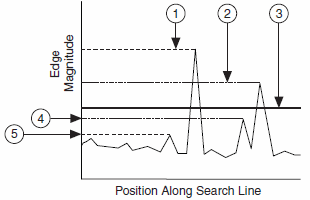1  Edge 1 Magnitude     2  Edge 2 Magnitude3  Threshold Level 4  Edge 2 Noise5  Edge 1 Noise

When looking for the best edge, the noise level is the strength of the second strongest edge before or after the detected edge, as shown in the following figure.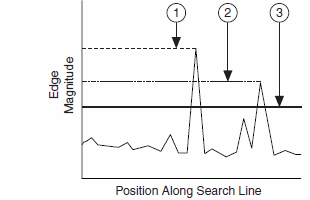1  Best Edge Magnitude     2  Best Edge Noise 3  Threshold Level

### Calibration Support for Edge Detection

The edge detection algorithm uses calibration information in the edge detection process if the original image is calibrated. For simple calibration, edge detection is performed directly on the image and the detected edge point locations are transformed into real-world coordinates. For perspective and non-linear distortion calibration, edge detection is performed on a corrected image. However, instead of correcting the entire image, only the area corresponding to the search region used for edge detection is corrected. Figure A and Figure B illustrate the edge detection process for calibrated images. Figure A shows an uncalibrated distorted image. Figure B shows the same image in a corrected image space.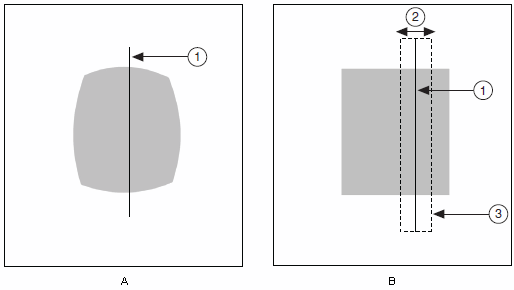1  Search Line     2  Search Width 3  Corrected Area

Information about the detected edge points is returned in both pixels and real-world units. Refer to system setup and calibration for more information about calibrating images.

## Extending Edge Detection to 2D Search Regions

The edge detection tool in NI Vision works on a 1D profile. The rake, spoke, and concentric rake tools extend the use of edge detection to two dimensions. In these edge detection variations, the 2D search area is covered by a number of search lines over which the edge detection is performed. You can control the number of the search lines used in the search region by defining the separation between the lines.

### Rake

A Rake works on a rectangular search region, along search lines that are drawn parallel to the orientation of the rectangle. Control the number of lines in the area by specifying the search direction as left to right or right to left for a horizontally oriented rectangle. Specify the search direction as top to bottom or bottom to top for a vertically oriented rectangle. The following figure illustrates the basics of the rake function.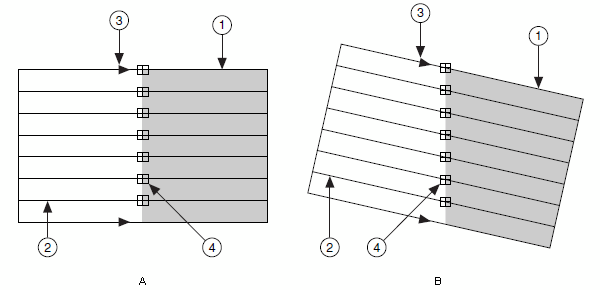1  Search Area     2  Search Line 3  Search Direction4  Edge Points

### Spoke

A Spoke works on an annular search region, along search lines that are drawn from the center of the region to the outer boundary and that fall within the search area. Control the number of lines in the region by specifying the angle between each line. Specify the search direction as either from the center outward or from the outer boundary to the center. The following figure illustrates the basics of the spoke function.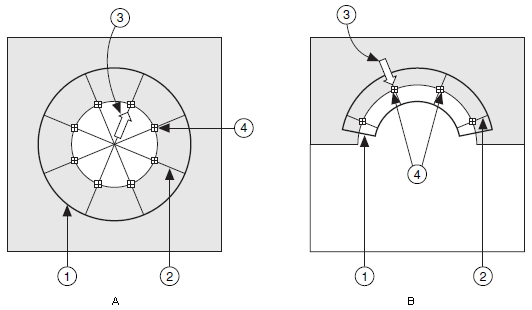1  Search Area     2  Search Line 3  Search Direction4  Edge Points

### Concentric Rake

A Concentric Rake works on an annular search region. It is an adaptation of the rake to an annular region. The following illustrates the basics of the concentric rake. Edge detection is performed along search lines that occur in the search region and that are concentric to the outer circular boundary. Control the number of concentric search lines that are used for the edge detection by specifying the radial distance between the concentric lines in pixels. Specify the direction of the search as either clockwise or anti-clockwise.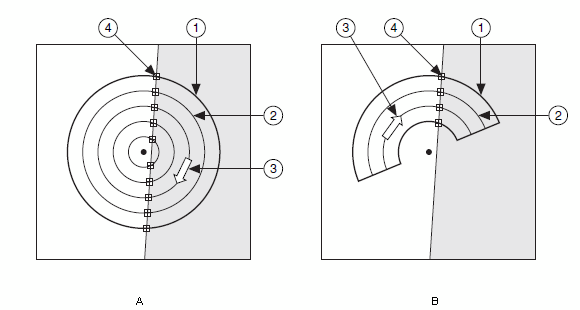1  Search Area     2  Search Line 3  Search Direction4  Edge Points

## Finding Straight Edges

Finding straight edges is another extension of edge detection to 2D search regions. Finding straight edges involves finding straight edges, or lines, in an image within a 2D search region. Straight edges are located by first locating 1D edge points in the search region and then computing the straight lines that best fit the detected edge points. Straight edge methods can be broadly classified into two distinct groups based on how the 1D edge points are detected in the image.

### Rake-Based Methods

A Rake is used to detect edge points within a rectangular search region. Straight lines are then fit to the edge points. Three different options are available to determine the edge points through which the straight lines are fit.

#### First Edges

A straight line is fit through the first edge point detected along each search line in the Rake. The method used to fit the straight line is described in dimensional measurements. The following figure shows an example of the straight edge detected on an object using the first dark to bright edges in the Rake along with the computed edge magnitudes along one search line in the Rake.

 Search Direction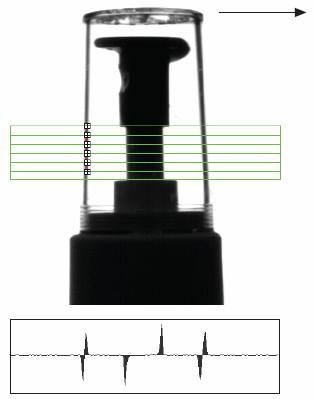#### Best Edges

A straight line is fit through the best edge point along each search line in the Rake. The method used to fit the straight line us described in dimensional measurements. The following figure shows an example of the straight edge detected on an object using the best dark to bright edges in the Rake along with the computed edge magnitudes along one search line in the Rake.

 Search Direction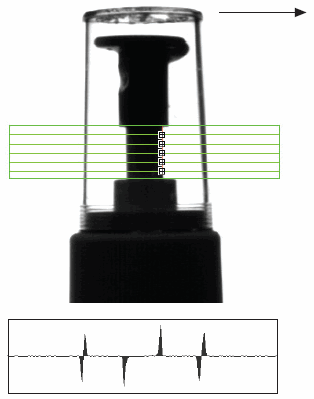#### Hough-Based Methods

In this method, a Hough transform is used to detect the straight edges, or lines, in an image. The Hough transform is a standard technique used in image analysis to find curves that can be parameterized, such as straight lines, polynomials, and circles. For detecting straight lines in an image, NI Vision uses the parameterized form of the line

ρ = xcosθ + ysinθ

where, ρ is the perpendicular distance from the origin to the line and θ is the angle of the normal from the origin to the line. Using this parameterization, a point (x, y) in the image is transformed into a sinusoidal curve in the (ρ, θ), or Hough space. The following figure illustrates the sinusoidal curves formed by three image points in the Hough space. The curves associated with colinear points in the image, intersect at a unique point in the Hough space. The coordinates (ρ, θ) of the intersection are used to define an equation for the corresponding line in the image. For example, the intersection point of the curves formed by points 1 and 2 represent the equation for Line1 in the image.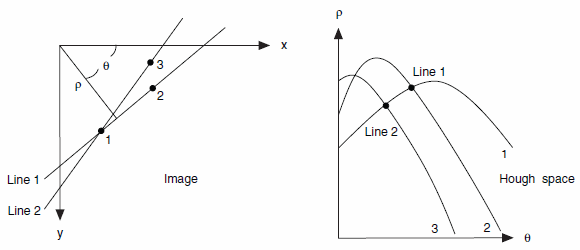The following figure illustrates how NI Vision uses the Hough transform to detect straight edges in an image. The location (x, y) of each detected edge point is mapped to a sinusoidal curve in the (ρ, θ) space. The Hough space is implemented as a two-dimensional histogram where the axes represent the quantized values for ρ and θ. The range for ρ is determined by the size of the search region, while the range for θ is determined by the angle range for straight lines to be detected in the image. Each edge location in the image maps to multiple locations in the Hough histogram, and the count at each location in the histogram is incremented by one. Locations in the histogram with a count of two or more correspond to intersection points between curves in the (ρ, θ) space. Figure B shows a two-dimensional image of the Hough histogram. The intensity of each pixel corresponds to the value of the histogram at that location. Locations where multiple curves intersect appear darker than other locations in the histogram. Darker pixels indicate stronger evidence for the presence of a straight edge in the original image because more points lie on the line. The following figure also shows the line formed by four edge points detected in the image and the corresponding intersection point in the Hough histogram.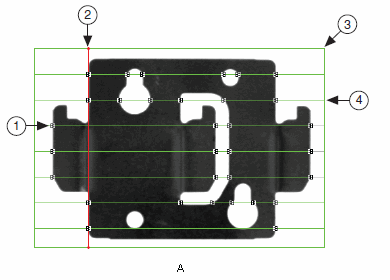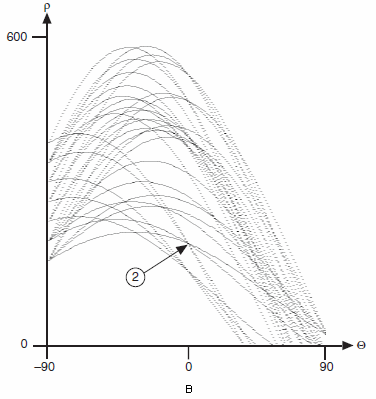1  Edge Point     2  Straight Edge 3  Search Region4  Search Line

Straight edges in the image are detected by identifying local maxima, or peaks in the Hough histogram. The local maxima are sorted in descending order based on the histogram count. To improve the computational speed of the straight edge detection process, only a few of the strongest peaks are considered as candidates for detected straight edges. For each candidate, a score is computed in the original image for the line that corresponds to the candidate. The line with the best score is returned as the straight edge. The Hough-based method also can be used to detect multiple straight edges in the original image. In this case, the straight edges are returned based on their scores.

### Projection-Based Methods

The projection-based method for detecting straight edges is an extension of the 1D edge detection process discussed in the advanced edge detection section. The following figure illustrates the projection-based straight edge detection process. The algorithm takes in a search region, search direction, and an angle range. The algorithm first either sums or finds the medians of the data in a direction perpendicular to the search direction. NI Vision then detects the edge position on the summed profile using the 1D edge detection function. The location of the edge peak is used to determine the location of the detected straight edge in the original image.

To detect the best straight edge within an angle range, the same process is repeated by rotating the search ROI through a specified angle range and using the strongest edge found to determine the location and angle of the straight edge.

 Search Direction SumPixels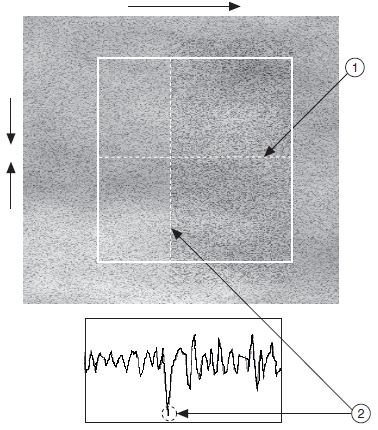1  Projection Axis      2  Best Edge Peak and Corresponding Line in the Image

The projection-based method is very effective for locating noisy and low-contrast straight edges.

The projection-based method also can detect multiple straight edges in the search region. For multiple straight edge detection, the strongest edge peak is computed for each point along the projection axis. This is done by rotating the search region through a specified angle range and computing the edge magnitudes at every angle for each point along the projection axis. The resulting peaks along the projection axis correspond to straight edges in the original image.

### Straight Edge Score

NI Vision returns an edge detection score for each straight edge detected in an image. The score ranges from 0 to 1000 and indicates the strength of the detected straight edge.

The edge detection score is defined as

 s c m + n
 where s is the edge detection score, c is the sum of the gradients at the edge points that match the specified edge polarity, m is the number of edge points on the straight line that match the specified edge polarity, and n is the number of edge points on the straight line that do not match the specified edge polarity.